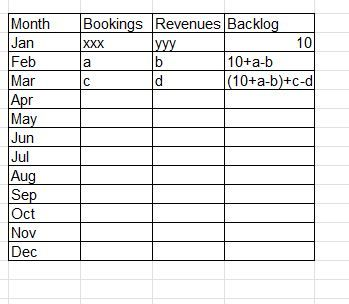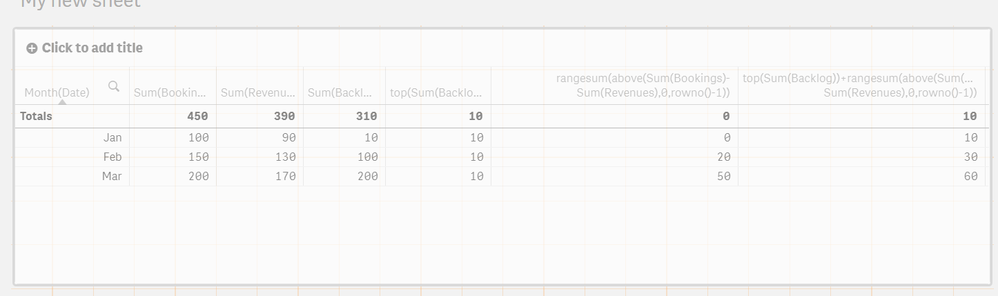# New to Qlik Sense

If you’re new to Qlik Sense, start with this Discussion Board and get up-to-speed quickly.

cancel
Showing results for
Did you mean:Creator

## Pivot table - Set analysis Recursion

Hey,

a bit of background:

each month we take a snapshot of our open backlog (that might have changed to due different changes in orders)

so, we would like to compare the snapshot to the "calculated" (that will take the first snapshot and + Bookings - Revenues)

i am looking to create the following table:to make it easier, i am even ok with setting the first month (jan) with a fixed number

the following calculation gives me the correct answer for the first row

If(Year=2021 and Month='Jan', SUM(Backlog),
Above(Sum(Backlog))+sum(Bookings)-sum( Revenues))

but after that i guess i need some kind of recursion or else it takes the Above(Sum(Backlog))

instead of the entire function

any ideas?

Many thanks,

Roi

1 Solution

Accepted SolutionsSpecialist II

Hi,

How about something like;

top(Sum(Backlog))+rangesum(above(Sum(Bookings)-Sum(Revenues),0,rowno()-1))

Shown in here with the parts broken out;Cheers,

Chris.

2 RepliesSpecialist II

Hi,

How about something like;

top(Sum(Backlog))+rangesum(above(Sum(Bookings)-Sum(Revenues),0,rowno()-1))

Shown in here with the parts broken out;Cheers,

Chris.Creator
Author

Thank you Chris! 🙂Tags
Community Browser